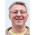## Pages

### Behaviour Driven Testing in C++

The basis of testing is very basic. You check that an expected value matches an actual value. For example, in JUnit we might write something like this...
```Wibble expected = someExpression;
Wibble actual = someOtherExpression;
assertEquals(expected, actual);
```
You might not have noticed it before but it's quite likely your test code contains a lot of repeated occurrences of that last line (in whatever form it takes). When something keeps re-occuring you should start to wonder what the missing abstraction is. The names expected and actual are extremely strong stylized hints that an assertEquals is about to happen. And then it does happen! So how about writing something like this instead?
```expected = 42;
actual = expression();
```
and somehow the test framework takes it as read that it has to do an assertEquals. In C++ we can put behaviour in a destructor and create an object in a scope, thus automating the behaviour when the object goes out of scope. Based on this idea I've come up with...
```ARE_EQUAL(int)
{
...
expected = 42;
actual = expression();
}
```
The idea is that ARE_EQUAL is a macro
```#define ARE_EQUAL(type) \
if (const wrapper<type> & expected = wrapper<type>()); \
else \
if (const wrapper<type> & actual = wrapper<type>()); \
else \
if (const auto_asserter<type> & rrid = \
auto_asserter<int>(expected,actual)); \
else
```
which relies on
```template<typename type>
struct wrapper
{
operator bool() const { return false; }
const wrapper & operator=(const type & rhs) const
{
value = rhs;
return *this;
}
mutable type value;
};
```
and
```template<typename type>
class auto_asserter
{
public:
auto_asserter(
const wrapper<type> & expected,
const wrapper<type> & actual)
: expected(expected)
, actual(actual)
{
}
~auto_asserter()
{
assert(expected.value == actual.value);
}
operator bool() const { return false; }
private:
const wrapper<type> & expected;
const wrapper<type> & actual;
};
```

#### 5 comments:

1.That is indeed neat and expresses what you're doing in the code clearly and literately.

However, in most cases I'd prefer to just write...

assertEquals(42, expression());

...unless expression() is too large and unweildy.

This is shorter, easier to read, and in a test file with many, many tests, less overwhelming to the reader.

Am I some kind of perverse deviant?

2.Agreed. It's really for situations when you have quite a lot to do before you get to the point where you can assign to expected and equals

3.And I should add that this very much a first cut. Something just to show the idea. It would need work to get better diagnostics if the assertion failed for example.

4.I like the extra scope '{}' that it introduces. Sometimes I've written the asserts for each overload* as part of the same test case which means numbering the variables (eg actual1, actual2 etc) or introducing dummy scopes which also looks ugly.

*I know, I know, I should be using long descriptive test names like Mr Henney suggests in "Programming with GUTs".

5.Yes a couple of people have asked about the scoping. When you write

if (type var = expression)
...
else
...

then var is in scope for the whole if statement, (the else part included) and only the if statement. So you can several of these constructs together and it works.